peteroznewman
Subscriber

Did you set Share Topology to Share so that the two bodies share nodes at the circular edge?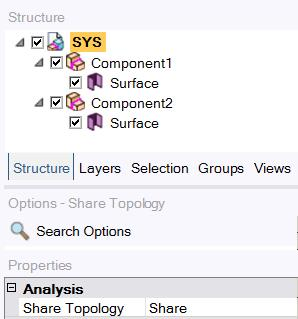When you do that, the mesh aligns.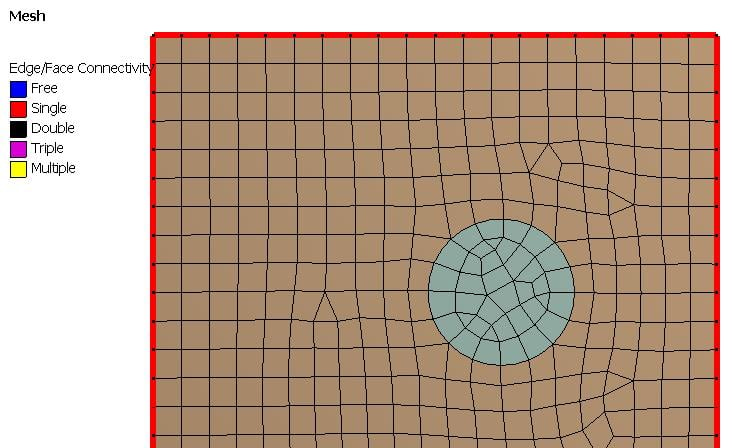If you have a left edge Temperature Boundary condition of 100 C, an internal heat generation, and a convection BC on the right edge, then heat will flow out of the left edge with the 100 C edge to maintain the 100 C.  You can see this if you plot Heat Flux. You can plot arrows of a fixed length to show direction, or you can plot the arrow length to be proportional to the magnitude of the heat flux so a point where the heat flux is large gets a long arrow and a point where the heat flux is small gets a short arrow.  The plots below have fixed length arrows.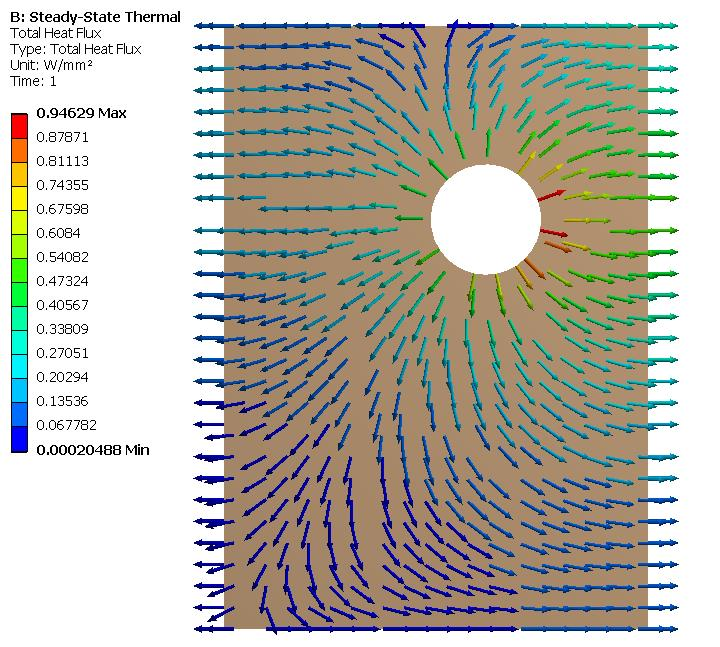If you make the left edge Temperature BC 500 C then for the SS solution, the heat will flow in from the left edge.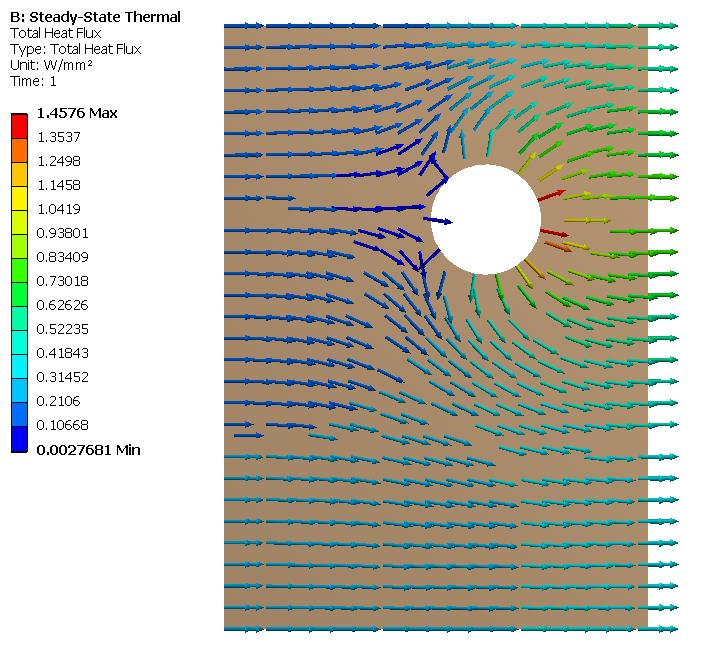And if you make the left edge Temperature BC 300 C, then the SS solution is the the heat flow changes direction from out to in on the left edge as you look from the top to the bottom.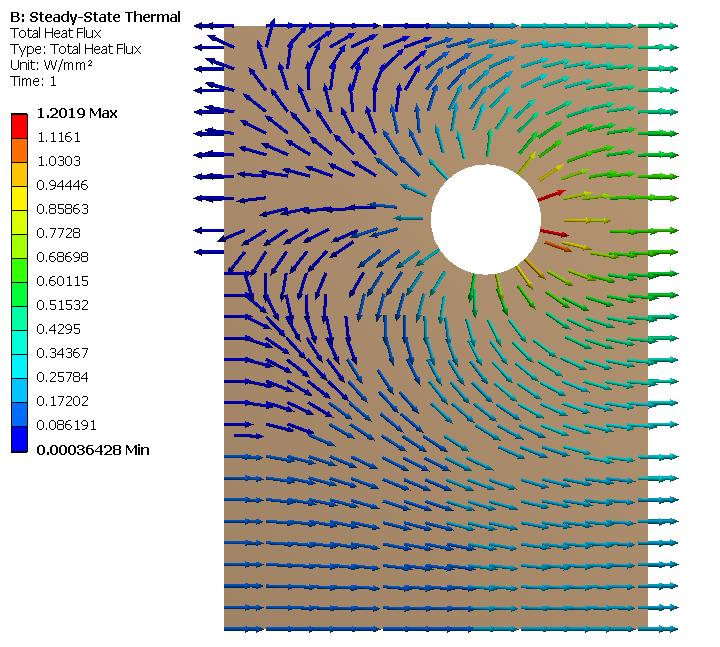The above were all Steady State Thermal analysis results. You should look at a Steady State result before you develop Transient models. For one thing, they solve a lot faster.  Why do you think the Transient solution is important?

3. Convection is a common BC because solid objects are usually touching some fluid, often air, that is at some bulk temperature, say room temperature of 22 C. Heat can flow out of a hot solid and into the room air by means of convection.  Or heat can flow into a cold solid from the warmer room air by means of convection. The convection film coefficient defines the heat flux as a function of the temperature difference between the solid and the air.